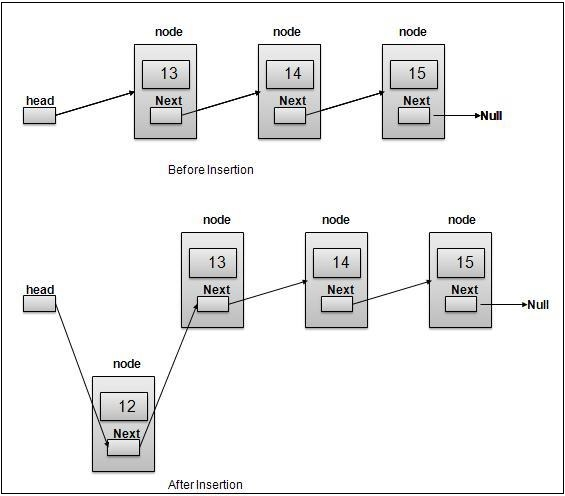# Inserting Elements to a doubly linked list using Javascript

We need to create a function insert(data, position) that inserts data at given position in the linked list. We'll perform the following steps −

• Create a new Node
• Check if the list is empty. If it then adds the node to head and tail and return.
• If not, then we'll iterate to the position we want to insert it to using currElem. We iterate a linked list by making currElem equal to currElem.next.

Now we change the links in the following way −

• Make new node point to next node in a list
• Make next node's previous point to the new node
• Make our node point to the previous node
• Make previous node's next point to the new node

Finally, we break the link from currElem to rest of the list and make it point to our created node. Now the node is in the list at the given position.

Here is an illustration of the same −Now let's have a look at how we'll implement this −

## Example

insert(data, position = this.length) {
let node = new this.Node(data);
this.length++;
// List is currently empty
this.tail = node;
}
if (position == 0) {
node.prev = null;
}
let iter = 1;
while (currNode.next != null && iter < position) {
currNode = currNode.next;
iter++;
}
// Make new node point to next node in list
node.next = currNode.next;
// Make next node's previous point to new node
if (currNode.next != null) {
currNode.next.prev = node;
}
// Make our node point to previous node
node.prev = currNode;
// Make previous node's next point to new node
currNode.next = node;
// check if inserted element was at the tail, if yes then make tail point to it
if (this.tail.next != null) {
this.tail = this.tail.next;
}
return node;
}

Note that we've given position as the last element. This is because if you don’t provide a position, it'll be inserted at the end by default.

You can test this using:

## Example

let list = new LinkedList();
list.insert(10);
list.insert(20);
list.insert(30);
list.insert(15, 2);
list.display();

## Output

This will give the output −

10 <->
30 <->
15 <->
20 <->

As we can see all the elements are in the order we intended. We tried inserting 15 at the position after 2.